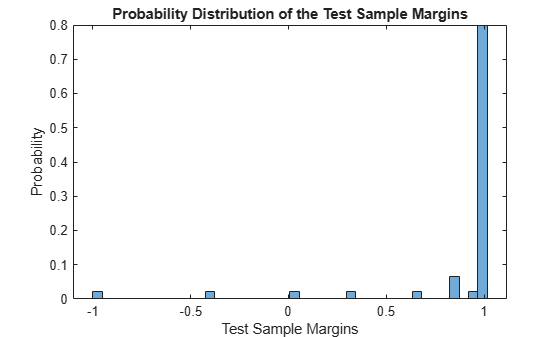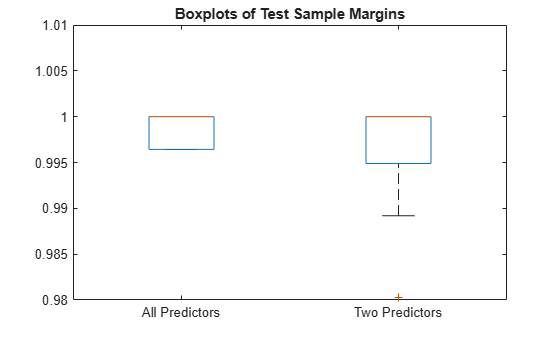# margin

Classification margins for naive Bayes classifier

## Syntax

``m = margin(Mdl,tbl,ResponseVarName)``
``m = margin(Mdl,tbl,Y)``
``m = margin(Mdl,X,Y)``

## Description

````m = margin(Mdl,tbl,ResponseVarName)` returns the Classification Margin (`m`) for the trained naive Bayes classifier `Mdl` using the predictor data in table `tbl` and the class labels in `tbl.ResponseVarName`.```
````m = margin(Mdl,tbl,Y)` returns the classification margins for `Mdl` using the predictor data in table `tbl` and the class labels in vector `Y`.```

example

````m = margin(Mdl,X,Y)` returns the classification margins for `Mdl` using the predictor data in matrix `X` and the class labels in `Y`.`m` is returned as a numeric vector with the same length as `Y`. The software estimates each entry of `m` using the trained naive Bayes classifier `Mdl`, the corresponding row of `X`, and the true class label `Y`.```

## Examples

collapse all

Estimate the test sample classification margins of a naive Bayes classifier. An observation margin is the observed true class score minus the maximum false class score among all scores in the respective class.

Load the `fisheriris` data set. Create `X` as a numeric matrix that contains four petal measurements for 150 irises. Create `Y` as a cell array of character vectors that contains the corresponding iris species.

```load fisheriris X = meas; Y = species; rng('default') % for reproducibility```

Randomly partition observations into a training set and a test set with stratification, using the class information in `Y`. Specify a 30% holdout sample for testing.

`cv = cvpartition(Y,'HoldOut',0.30);`

Extract the training and test indices.

```trainInds = training(cv); testInds = test(cv);```

Specify the training and test data sets.

```XTrain = X(trainInds,:); YTrain = Y(trainInds); XTest = X(testInds,:); YTest = Y(testInds);```

Train a naive Bayes classifier using the predictors `XTrain` and class labels `YTrain`. A recommended practice is to specify the class names. `fitcnb` assumes that each predictor is conditionally and normally distributed.

`Mdl = fitcnb(XTrain,YTrain,'ClassNames',{'setosa','versicolor','virginica'})`
```Mdl = ClassificationNaiveBayes ResponseName: 'Y' CategoricalPredictors: [] ClassNames: {'setosa' 'versicolor' 'virginica'} ScoreTransform: 'none' NumObservations: 105 DistributionNames: {'normal' 'normal' 'normal' 'normal'} DistributionParameters: {3x4 cell} Properties, Methods ```

`Mdl` is a trained `ClassificationNaiveBayes` classifier.

Estimate the test sample classification margins.

```m = margin(Mdl,XTest,YTest); median(m)```
```ans = 1.0000 ```

Display the histogram of the test sample classification margins.

```histogram(m,length(unique(m)),'Normalization','probability') xlabel('Test Sample Margins') ylabel('Probability') title('Probability Distribution of the Test Sample Margins')```Classifiers that yield relatively large margins are preferred.

Perform feature selection by comparing test sample margins from multiple models. Based solely on this comparison, the classifier with the highest margins is the best model.

Load the `fisheriris` data set. Specify the predictors `X` and class labels `Y`.

```load fisheriris X = meas; Y = species; rng('default') % for reproducibility```

Randomly partition observations into a training set and a test set with stratification, using the class information in `Y`. Specify a 30% holdout sample for testing. `Partition` defines the data set partition.

`cv = cvpartition(Y,'Holdout',0.30);`

Extract the training and test indices.

```trainInds = training(cv); testInds = test(cv);```

Specify the training and test data sets.

```XTrain = X(trainInds,:); YTrain = Y(trainInds); XTest = X(testInds,:); YTest = Y(testInds);```

Define these two data sets:

• `fullX` contains all predictors.

• `partX` contains the last two predictors.

```fullX = XTrain; partX = XTrain(:,3:4);```

Train a naive Bayes classifier for each predictor set.

```fullMdl = fitcnb(fullX,YTrain); partMdl = fitcnb(partX,YTrain);```

`fullMdl` and p`artMdl` are trained `ClassificationNaiveBayes` classifiers.

Estimate the test sample margins for each classifier.

```fullM = margin(fullMdl,XTest,YTest); median(fullM)```
```ans = 1.0000 ```
```partM = margin(partMdl,XTest(:,3:4),YTest); median(partM)```
```ans = 1.0000 ```

Display the distribution of the margins for each model using boxplots.

```boxplot([fullM partM],'Labels',{'All Predictors','Two Predictors'}) ylim([0.98 1.01]) % Modify the y-axis limits to see the boxes title('Boxplots of Test Sample Margins')```The margins for `fullMdl` (all predictors model) and `partMdl` (two predictors model) have a similar distribution with the same median. `partMdl` is less complex but has outliers.

## Input Arguments

collapse all

Naive Bayes classification model, specified as a `ClassificationNaiveBayes` model object or `CompactClassificationNaiveBayes` model object returned by `fitcnb` or `compact`, respectively.

Sample data used to train the model, specified as a table. Each row of `tbl` corresponds to one observation, and each column corresponds to one predictor variable. `tbl` must contain all the predictors used to train `Mdl`. Multicolumn variables and cell arrays other than cell arrays of character vectors are not allowed. Optionally, `tbl` can contain additional columns for the response variable and observation weights.

If you train `Mdl` using sample data contained in a table, then the input data for `margin` must also be in a table.

Response variable name, specified as the name of a variable in `tbl`.

You must specify `ResponseVarName` as a character vector or string scalar. For example, if the response variable `y` is stored as `tbl.y`, then specify it as `'y'`. Otherwise, the software treats all columns of `tbl`, including `y`, as predictors.

If `tbl` contains the response variable used to train `Mdl`, then you do not need to specify `ResponseVarName`.

The response variable must be a categorical, character, or string array, logical or numeric vector, or cell array of character vectors. If the response variable is a character array, then each element must correspond to one row of the array.

Data Types: `char` | `string`

Predictor data, specified as a numeric matrix.

Each row of `X` corresponds to one observation (also known as an instance or example), and each column corresponds to one variable (also known as a feature). The variables in the columns of `X` must be the same as the variables that trained the `Mdl` classifier.

The length of `Y` and the number of rows of `X` must be equal.

Data Types: `double` | `single`

Class labels, specified as a categorical, character, or string array, logical or numeric vector, or cell array of character vectors. `Y` must have the same data type as `Mdl.ClassNames`. (The software treats string arrays as cell arrays of character vectors.)

The length of `Y` must be equal to the number of rows of `tbl` or `X`.

Data Types: `categorical` | `char` | `string` | `logical` | `single` | `double` | `cell`

collapse all

### Classification Edge

The classification edge is the weighted mean of the classification margins.

If you supply weights, then the software normalizes them to sum to the prior probability of their respective class. The software uses the normalized weights to compute the weighted mean.

When choosing among multiple classifiers to perform a task such as feature section, choose the classifier that yields the highest edge.

### Classification Margin

The classification margin for each observation is the difference between the score for the true class and the maximal score for the false classes. Margins provide a classification confidence measure; among multiple classifiers, those that yield larger margins (on the same scale) are better.

### Posterior Probability

The posterior probability is the probability that an observation belongs in a particular class, given the data.

For naive Bayes, the posterior probability that a classification is k for a given observation (x1,...,xP) is

`$\stackrel{^}{P}\left(Y=k|{x}_{1},..,{x}_{P}\right)=\frac{P\left({X}_{1},...,{X}_{P}|y=k\right)\pi \left(Y=k\right)}{P\left({X}_{1},...,{X}_{P}\right)},$`

where:

• $P\left({X}_{1},...,{X}_{P}|y=k\right)$ is the conditional joint density of the predictors given they are in class k. `Mdl.DistributionNames` stores the distribution names of the predictors.

• π(Y = k) is the class prior probability distribution. `Mdl.Prior` stores the prior distribution.

• $P\left({X}_{1},..,{X}_{P}\right)$ is the joint density of the predictors. The classes are discrete, so $P\left({X}_{1},...,{X}_{P}\right)=\sum _{k=1}^{K}P\left({X}_{1},...,{X}_{P}|y=k\right)\pi \left(Y=k\right).$

### Prior Probability

The prior probability of a class is the assumed relative frequency with which observations from that class occur in a population.

### Score

The naive Bayes score is the class posterior probability given the observation.